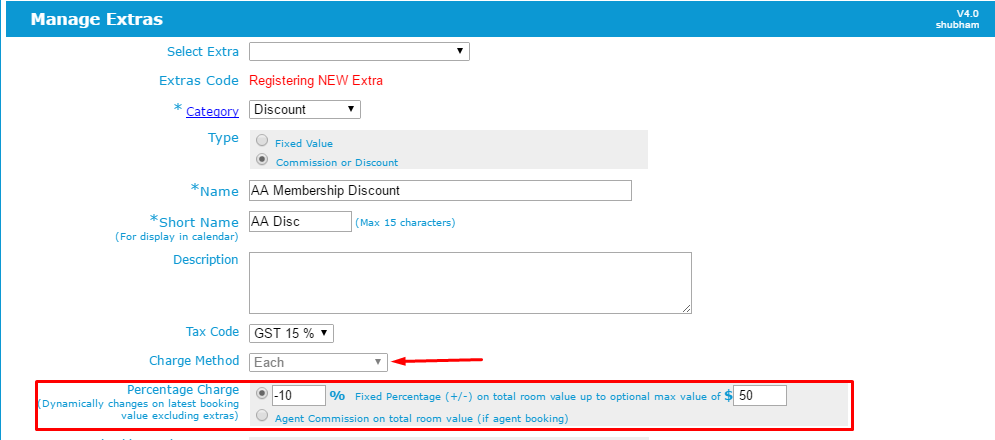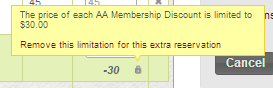### Percentage Based Extras

You can set an extra to be charged a fixed percentage of the value of the room value of the booking.

Once set, iBex will calculate the price to charge (or discount) depending on the percentage set. You can also set a maximum value so if the price calculated is more than the maximum amount set, only the maximum set will be applied.

An example of when you may use this is if you have a Loyalty Scheme where members receive a % discount with a maximum discount allowed. Since the discount applies to the room value, if they extend their stay or change rooms the extra will automatically recalculate the correct charge.

Another example of when you may use this is if you have agents who make direct bookings for you and want to see their commission on their booking receipt (rather than being invoiced). As above, if the room value changes for any reason, then the extra will automatically be recalculated.

This extra can be applied to the whole booking or individual rooms.

The price calculated is the percentage of the room value only – not the booking value.1. When you are setting up a new extra, set the Charge Method to Each 2. In the Percentage Charge field, either enter the amount required and the maximum value if applicable, or select Agent Commission if you are using this extra to log your agents or sales channels commission amount.

The value entered must be negative if this is a refund or discount, or a positive value if this is a charge.

3. Continue to set up the extra as necessary.

4. Once saved, do not add any price values as iBex will automatically calculate the amount when it is saved to the booking.

Once the extra has been registered, you can add the extra to the booking and apply it to the whole booking or to individual rooms. This is useful if you have a group booking where only one of the guests requires the extra.

Please see below for an example of how to add the extra to a booking...

• Go to the booking
• Go to the Extras tab
• If extras are already in the booking, click the Add or Edit Extras button
• Select who to charge the extra to
• Select the extra in the Extra column
• Update the Title if required and check the quantity and dates
• You will see the suggested amount being the percentage that you have set for the extra

iBex will add the percentage amount as the Rate with the actual amount calculated below the rate field (see the example above). The amount charged will be the amount shown. If you want to change the percentage, you can change it in the rate field and iBex will update the amount that it equals so that you can set the percentage at the amount that you want.

You will notice that there is a padlock next to the amount to be charged (see the example above in the red square). This will appear if you have set a maximum amount to be charged in the extra’s settings (as shown in the extra set up example above). If you want to override this setting and increase the value beyond the maximum set, hover over the padlock and a pop up will appear where you can remove the limitation for this extra.

If you click on ‘Remove this limitation for this extra reservation’, the padlock will become unlocked and the true amount for the percentage set will then be calculated and charged. To put the limit back on, just do the same.

iBex will add the percentage amount as the Rate with the actual amount calculated below the rate field (see the example above). The amount charged will be the amount shown. If you want to change the percentage, you can change it in the rate field and iBex will update the amount that it equals so that you can set the percentage at the amount that you want.

You will notice that there is a padlock next to the amount to be charged (see the example above in the red square). This will appear if you have set a maximum amount to be charged in the extra’s settings (as shown in the extra set up example above). If you want to override this setting and increase the value beyond the maximum set, hover over the padlock and a pop up will appear where you can remove the limitation for this extra.

If you click on ‘Remove this limitation for this extra reservation’, the padlock will become unlocked and the true amount for the percentage set will then be calculated and charged. To put the limit back on, just do the same.